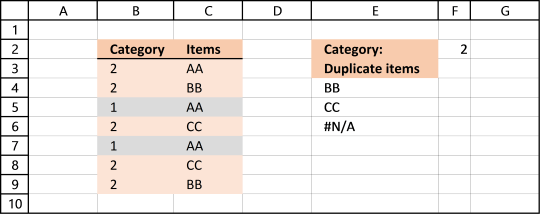Author: Oscar Cronquist Article last updated on August 19, 2017

Question: How do I filter duplicates with a condition?

Column B contains category and column C contains Items. Only duplicate Items with adjacent Category number 2 is listed in column E.AA is in category 2 (row 3) and 1 but exists only once in category 2. It is not a duplicate.

BB is in category 2 and exists twice (row 4 and 9). BB has a duplicate.

CC is in category 2 and has a duplicate (row 6 and 8). CC is a duplicate.

Formula in cell E4:

=LOOKUP(2, 1/(((COUNTIF(\$E\$3:E3, \$C\$3:\$C\$9)=0)*(COUNTIFS(\$B\$3:\$B\$9, \$F\$2, \$C\$3:\$C\$9, \$C\$3:\$C\$9)>1))), \$C\$3:\$C\$9)

Extract a list of alphabetically sorted duplicates from a column

The following array formula extracts duplicate values sorted from A to Z from cell range B3:B21. Excel array formula in […]

Learn how to filter duplicates:

Extract a list of duplicates from a column

The array formula in cell C2 extracts duplicate values from column A. Only one duplicate of each value is displayed […]

You can also use more than one condition, this article shows you how:

Filter duplicate values using critera

The following worksheet allows you to search for duplicate names using a date range (cell B1 and B2) and a […]

Filter a unique distinct list:

5 easy ways to extract Unique Distinct Values

First, let me explain the difference between unique values and unique distinct values, it is important you know the difference […]

### Explaining formula in cell E4

Step 1 - Identify values that has not been displayed before

COUNTIF(\$E\$3:E3, \$C\$3:\$C\$9)=0

becomes

COUNTIF("Duplicate items",{"AA";"BB";"AA";"CC";"AA";"CC";"BB"})=0

and returns

{TRUE;TRUE;TRUE;TRUE;TRUE;TRUE;TRUE}

In cell E4 no values has been shown before so the array returns TRUE for all values.

How to use the COUNTIF function

Counts the number of cells that meet a specific condition.

Step 2 - Find duplicates in category 2

COUNTIFS(\$B\$3:\$B\$9, \$F\$2, \$C\$3:\$C\$9, \$C\$3:\$C\$9)>1

becomes

COUNTIFS({2;2; 1;2;1; 2;2}, 2, {"AA";"BB";"AA";"CC";"AA";"CC";"BB"}, {"AA";"BB";"AA";"CC";"AA";"CC";"BB"})>1

becomes

{1;2;1;2;1;2;2}>1

and returns {FALSE;TRUE; FALSE;TRUE;FALSE; TRUE;TRUE}

How to use the COUNTIFS function

Checks multiple conditions against the same number of cell ranges and counts how many times all criteria are met.

Step 3 - Multiply arrays

(COUNTIF(\$E\$3:E3, \$C\$3:\$C\$9)=0)*(COUNTIFS(\$B\$3:\$B\$9, \$F\$2, \$C\$3:\$C\$9, \$C\$3:\$C\$9)>1)

becomes

{TRUE;TRUE;TRUE;TRUE;TRUE;TRUE;TRUE} * {FALSE;TRUE; FALSE;TRUE;FALSE; TRUE;TRUE}

and returns {0;1; 0;1;0; 1;1}

Step 4 - Divide 1 with array

1/(((COUNTIF(\$E\$3:E3, \$C\$3:\$C\$9)=0)*(COUNTIFS(\$B\$3:\$B\$9, \$F\$2, \$C\$3:\$C\$9, \$C\$3:\$C\$9)>1)))

becomes

1/{0;1; 0;1;0; 1;1}

and returns {#DIV/0!; 1;#DIV/0!; 1;#DIV/0!; 1;1}

Step 5 - Find last matching value in array

The LOOKUP function ignores errors but requires the second argument to be sorted ascending. However our list contains only errors or 1 so the LOOKUP function returns the last matching 1 in the array.

LOOKUP(2, 1/(((COUNTIF(\$E\$3:E3, \$C\$3:\$C\$9)=0)*(COUNTIFS(\$B\$3:\$B\$9, \$F\$2, \$C\$3:\$C\$9, \$C\$3:\$C\$9)>1))), \$C\$3:\$C\$9)

becomes

LOOKUP(2, {#DIV/0!; 1;#DIV/0!; 1;#DIV/0!; 1;1}, \$C\$3:\$C\$9)

becomes

LOOKUP(2, {#DIV/0!; 1;#DIV/0!; 1;#DIV/0!; 1;1}, {"AA";"BB";"AA";"CC";"AA";"CC";"BB"})

and returns "BB" in cell E4. BB is the corresponding value to the last 1 in the array, bolded above.

How to use the LOOKUP function

Finds a value in a sorted cell range and returns a value on the same row.

Step 6 - Relative cell refences

The following cell reference is both an absolute and relative cell reference: \$E\$3:E3. When you copy the formula to cells below, the cell ref changes. That way the formula knows which values have been displayed before.

How to use absolute and relative references

What is a reference in Excel? Excel has an A1 reference style meaning columns are named letters A to XFD […]